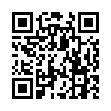# North Coast Synthesis Ltd.

## F♭+2+4+♯2Choose audio sample set:
MSK 007 Leapfrog VCF (patch; see in online store)
MSK 009 Coiler VCF (patch; see in online store)

Equivalent chord symbols: E+2+4+♯2, E+4+9+♯2, E+2+11+♯2, F♭+4+9+♯2, F♭+2+11+♯2.

Notes in this chord: F♭, G♭, G, A♭, B♭♭, C♭. Integer notation: {4, 6, 7, 8, 9, 11}.

Nearby chords (one less note): E+2+4, E+2+♯2, E+4+♯2, Em+2+4, E4+2+♯2, G♭m4+2+♯1.

Nearby chords (one more note): E11+♯2, EM11+♯2, D13-1+♯4, G♭m11+♯1, E+2+4+♯1+♯2, E+2+4+♯2+♯4.

Parallel chords (same structure, different root): C+2+4+♯2, D+2+4+♯2, E+2+4+♯2, F+2+4+♯2, G+2+4+♯2, A+2+4+♯2, B+2+4+♯2, C♭+2+4+♯2, D♭+2+4+♯2, E♭+2+4+♯2, G♭+2+4+♯2, A♭+2+4+♯2, B♭+2+4+♯2, C♯+2+4+♯2, D♯+2+4+♯2, E♯+2+4+♯2, F♯+2+4+♯2, G♯+2+4+♯2, A♯+2+4+♯2, B♯+2+4+♯2.

Experimental fretting charts for guitar standard EADGBE tuning (change tuning or instrument):